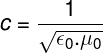Optics

# Speed ​​of light

The speed of light in a vacuum is an important physical constant whose value is approximately 300 million meters per second.

The speed of light , commonly denoted by the letter c, is about 299,792,458 m/s , that is, every second, light travels approximately 300,000 km when propagating in a vacuum. The choice of letter c is due to the Latin word celeritas , which means speed.

The speed of light is used to define the meter, a unit of length used by the International System of Units (SI) as the measure of the space traveled by light in a vacuum in a time of 1/299,792,458 second.

## Who measured the speed of light for the first time?

The speed of propagation of light was a question of scientists since the oldest civilizations. Empedocles argued that light had infinite speed. Ptolemy and Euclid believed that it was emitted by the eyes, and other great Greek philosophers and mathematicians, such as Aristotle and Hero of Alexandria, believed that light propagated through bodies, through space, with finite speed . These views influenced the thinking of great scientists such as Johannes Kepler (1571-1630) and René Descartes .

In 1638, Galileo Galilei (1564-1642) even carried out an experiment in order to calculate the speed of light. To do this, he measured the time it took her to leave a flashlight on top of a mountain and arrive at another 2 kilometers away. His conclusions pointed out that the time for light to travel this distance was less than 10 -5 s (0.00001 s), a very small interval to be measured with the technological resources available at that time.

A short time later, in 1676, the Danish astronomer Ole Romer observed that the duration of eclipses of Jupiter’s moons varied according to the time of year. Romer suspected that the variation was due to light having finite speed, considering that the distance from Earth to Jupiter’s moons changes according to their orbits.

It was not until 1819 that an experiment was able to provide accurate measurements of the speed of light. By means of a semi-reflecting mirror and a rotating cog wheel, the French physicist Armand Hyppolyte Fizeau managed to define the speed of light, with an error slightly less than 10% in relation to the current measurement.

Fizeau ‘s experiment worked like this:

1. The light was emitted by a light source L. This light was converged through a convex lens and directed towards a semi -reflecting mirror P, with an angle of 45º in relation to the light rays. Part of these rays of light passed through the mirror and part of them was reflected ;

2. The part of the rays that was reflected passed through a rotating sprocket This light was reflected to a mirror S , about 8 km ahead, and back. Fizeau regulated the rotation speed of his wheel, since the reflected light took a short time to return to the semi-reflecting mirror P, until it was no longer possible to see the image reflected by the mirror S. first mirror.

## What does the speed of light depend on?

The speed of light depends on a property of optical media called refringence. The more refractive a medium, the slower the propagation speed of light inside it. Light undergoes refraction as it travels through some medium other than vacuum, and the measure of its refraction is called the index of refraction .

The refractive index is a measure of how much the speed of light in a given medium has been reduced relative to its speed in vacuum. It is therefore defined as the ratio of the speed of light in the medium ( v ) to the speed of light in vacuum ( c ).

We can take the diamond as an example: its refractive index is 2.4, which means that the speed of light in a vacuum is 2.4 times faster than its propagation inside the diamond.

Through the union of the Electricity and Magnetism equations, James Clerk Maxwell managed to develop the Electromagnetism equations that describe light as a transverse electromagnetic wave. Through his calculations, Maxwell was able to determine the speed of light based on even more fundamental physical constants: the electrical and magnetic constants of the vacuum, called the permittivity (ε 0 ) and permeability (μ 0 ) of the vacuum. In this way, the speed of light can be obtained, through these two constants, by the expression: